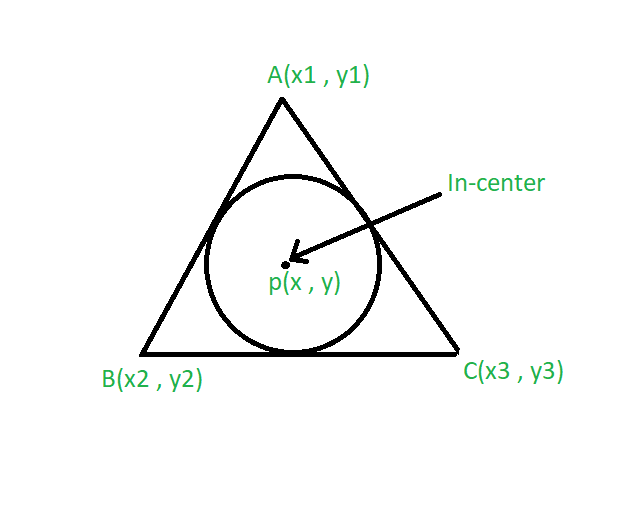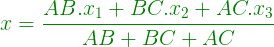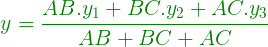Open In App

# Program to Find the Incenter of a Triangle

Given the vertices of a triangle and length of its sides. A circle is inscribed in a triangle. The task is to find the incenter of a triangle.
Examples:

Input: A(2, 2), B(1, 1), C(3, 1)
Output: (2, 1.5)

Input: A(3, 3), B(1, 2), C(2, 2)
Output: (2.5, 2.83)Approach:

• The center of the circle that touches the sides of a triangle is called its incenter.
• Suppose the vertices of the triangle are A(x1, y1), B(x2, y2) and C(x3, y3).
• Let the side AB = a, BC = b, AC = c then the coordinates of the in-center is given by the formula:••

Below is the implementation of the above approach:

## C++

 `// C++ program to find the``// incenter of a triangle``#include ``using` `namespace` `std;` `// Driver code``int` `main()``{``    ``// coordinate of the vertices``    ``float` `x1 = 2, x2 = 1, x3 = 3;``    ``float` `y1 = 2, y2 = 1, y3 = 1;``    ``float` `a = 2, b = 1, c = 1;` `    ``// Formula to calculate in-center``    ``float` `x = (a * x1 + b *``                   ``x2 + c * x3) / (a + b + c);``    ``float` `y = (a * y1 + b *``                   ``y2 + c * y3) / (a + b + c);` `    ``// System.out.print(setprecision(3));``    ``cout << ``"Incenter = "``         ``<< ``"("` `<< x << ``", "` `<< y << ``")"``;``    ``return` `0;``}` `// This code is contributed by 29AjayKumar`

## Java

 `// Java program to find the``// incenter of a triangle` `import` `java.util.*;``import` `java.lang.*;` `class` `GFG {` `    ``// Driver code``    ``public` `static` `void` `main(String args[])``    ``{``        ``// coordinate of the vertices``        ``float` `x1 = ``2``, x2 = ``1``, x3 = ``3``;``        ``float` `y1 = ``2``, y2 = ``1``, y3 = ``1``;``        ``float` `a = ``2``, b = ``1``, c = ``1``;` `        ``// Formula to calculate in-center``        ``float` `x``            ``= (a * x1 + b * x2 + c * x3) / (a + b + c);``        ``float` `y``            ``= (a * y1 + b * y2 + c * y3) / (a + b + c);` `        ``// System.out.print(setprecision(3));``        ``System.out.println(``"Incenter= "``                           ``+ ``"("` `+ x + ``", "` `+ y + ``")"``);``    ``}``}`

## Python3

 `# Python3 program to find the``# incenter of a triangle` `# Driver code` `# coordinate of the vertices``x1 ``=` `2``; x2 ``=` `1``; x3 ``=` `3``;``y1 ``=` `2``; y2 ``=` `1``; y3 ``=` `1``;``a ``=` `2``; b ``=` `1``; c ``=` `1``;` `# Formula to calculate in-center``x ``=` `(a ``*` `x1 ``+` `b ``*` `x2 ``+` `c ``*` `x3) ``/` `(a ``+` `b ``+` `c);``y ``=` `(a ``*` `y1 ``+` `b ``*` `y2 ``+` `c ``*` `y3) ``/` `(a ``+` `b ``+` `c);` `# System.out.print(setprecision(3));``print``(``"Incenter = ("``, x, ``","``, y, ``")"``);` `# This code is contributed``# by Akanksha Rai`

## C#

 `// C# program to find the``// incenter of a triangle` `using` `System;` `class` `GFG``{` `    ``// Driver code``    ``public` `static` `void` `Main()``    ``{``        ``// coordinate of the vertices``        ``float` `x1 = 2, x2 = 1, x3 = 3;``        ``float` `y1 = 2, y2 = 1, y3 = 1;``        ``float` `a = 2, b = 1, c = 1;` `        ``// Formula to calculate in-center``        ``float` `x``            ``= (a * x1 + b * x2 + c * x3) / (a + b + c);``        ``float` `y``            ``= (a * y1 + b * y2 + c * y3) / (a + b + c);` `        ``// System.out.print(setprecision(3));``        ``Console.WriteLine(``"Incenter= "``                        ``+ ``"("` `+ x + ``", "` `+ y + ``")"``);``    ``}``}` `// This code is contributed by vt_m.`

## Javascript

 ``

Output

`Incenter = (2, 1.5)`

Time Complexity: O(1), the code will run in a constant time.
Auxiliary Space: O(1), no extra space is required, so it is a constant.